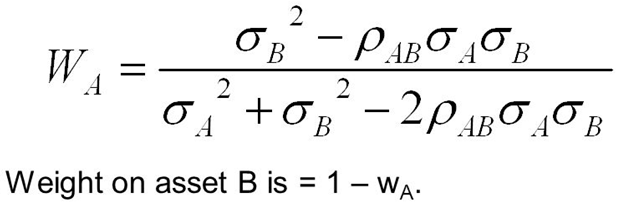# An investor can design a risky portfolio based on two stocks, A and B. The standard deviation of return on stock A is 20%, while the standard deviation on stock B is 15%. The correlation coefficient between the returns on A and B is 0%. The rate of return for stocks A and B is 20 and 10 respectively. The expected return on the minimum-variance portfolio is approximately _________.

Question
11 views

An investor can design a risky portfolio based on two stocks, A and B. The standard deviation of return on stock A is 20%, while the standard deviation on stock B is 15%. The correlation coefficient between the returns on A and B is 0%. The rate of return for stocks A and B is 20 and 10 respectively. The expected return on the minimum-variance portfolio is approximately _________.

check_circle

Step 1

The weights of assets A & B in minimum variance portfolio is given by the expressions below. The symbols have their usual meanings in the world of investment portfolio management:Step 2

WA = (0.152 - 0 x 0.20 x 0.15) / (0.202 + 0.152 - 2 x 0 x 0.20 x 0.15) = 0.36 = 36%

WB ...

### Want to see the full answer?

See Solution

#### Want to see this answer and more?

Solutions are written by subject experts who are available 24/7. Questions are typically answered within 1 hour.*

See Solution
*Response times may vary by subject and question.
Tagged in